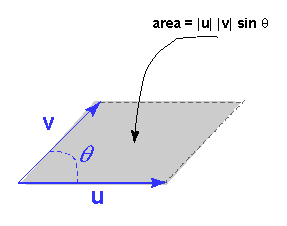No. The two possible cross products between u and v point in opposite directions.

# Visualizing the Cross ProductThe picture shows two vectors u and v and the parallelogram they define between them.

The area of the parallelogram is: | u | | v | sin θ where θ is the enclosed angle.

This area is the same as the magnitude of the cross product. This fact is sometimes helpful in visualizing the cross product. For example, if u is rotated so that its orientation approaches that of v (but its length is not changed), the area of the parallelogram gets close to zero, as does the magnitude of the cross product.

### QUESTION 5:

What angle between u and v will maximize their cross product? (Hint: look at the diagram)